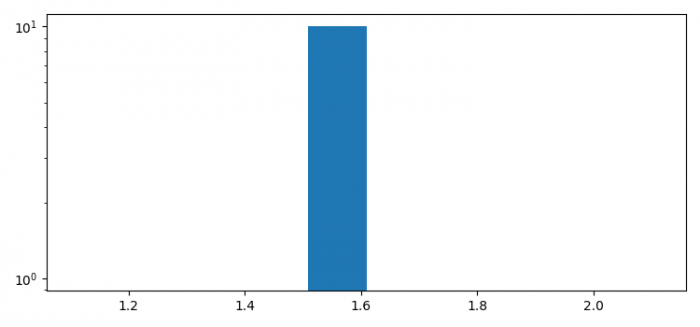# How to make a log histogram in Python?

To make a log histogram, we can use log=True in the argument of the hist() method.

## Steps

• Make a list of numbers.

• Plot a histogram with density=True.

• To display the figure, use show() method.

## Example

import numpy as np
import matplotlib.pyplot as plt
plt.rcParams["figure.figsize"] = [7.50, 3.50]
plt.rcParams["figure.autolayout"] = True
k = np.array([5, 5, 5, 5])
x, bins, p = plt.hist(np.log(k), density=True, log=True)
plt.show()

## OutputUpdated on: 07-May-2021

2K+ Views